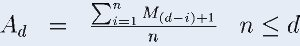#Simple Moving Average Calculator

simply calculating the average of a certain number of periods upto that point in time.

# Results...

Average for DayAverage Value
A33
A44
A55
A66.333
A78## Formula to Calculate Simple Moving Average (SMA)

A moving average is a calculation to analyze data points by creating a series of averages of different subsets of the full data set.Where,

n is number of data.

d is moving average.

Days M is Data